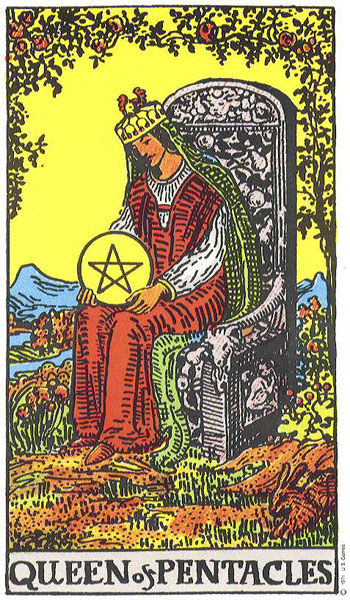## people with a 66 (Queen of Pentacles) life lesson

### March 5, 1970people with a 66 life lesson:Sabeer Bhatia

born December 30th, 1968

12 + 30 +1+9+6+8 = 66 = Bringing home the bacon.  Managing money.

Russell Wilson

born November 29th, 1988                                  11 + 29 +1+9+8+8 = 66

Laila Ali

born December 30th, 1977                                   12 + 30 +1+9+7+7 = 66

Ellie Goulding

born December 30th, 1986                                   12 + 30 +1+9+8+6 = 66

Ryleigh Vertes

born December 30th, 1995                                    12 + 30 +1+9+9+5 = 66

Kim Taehyung

born December 30th, 1995                                    12 + 30 +1+9+9+5 = 66

Joshua Hong

born December 30th, 1995                                    12 + 30 +1+9+9+5 = 66

Bebe Neuwirth

born December 31st, 1958                                      12 + 31 +1+9+5+8 = 66

Jennifer Ehle

born December 29th, 1969                                     12 + 29 +1+9+6+9 = 66

C. S. Lewis

born November 29th, 1898                                     11 + 29 +1+8+9+8 = 66